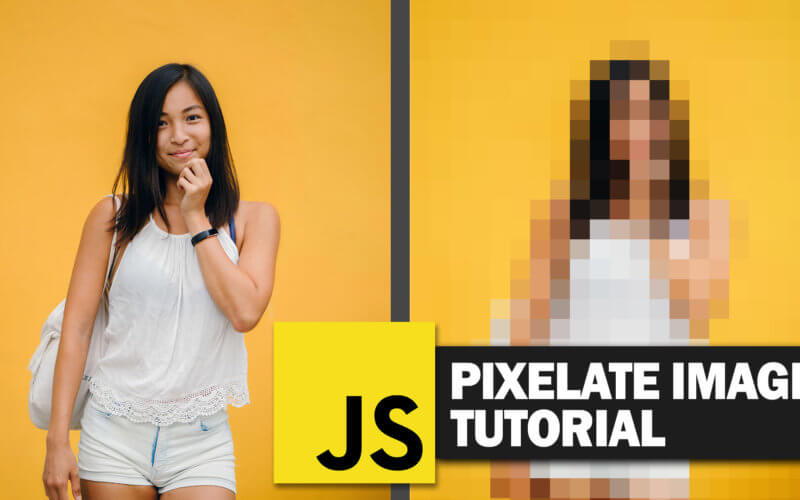# How to create pixelated image with JavaScript

In this tutorial, we’ll show you how to create pixelated image (aka. mosaic/censor) with JavaScript and canvas

So here is the example page. There is only one image on it and nothing else except a few CSS rules for center alignment.

`<img id="image1" src="image1.jpg" />`
```body {
margin: 0;
width: 100vw;
height:100vh;
display:flex;
align-items: center;
justify-content: center;
}
#image1 {
width: 800px;
height: auto;
}```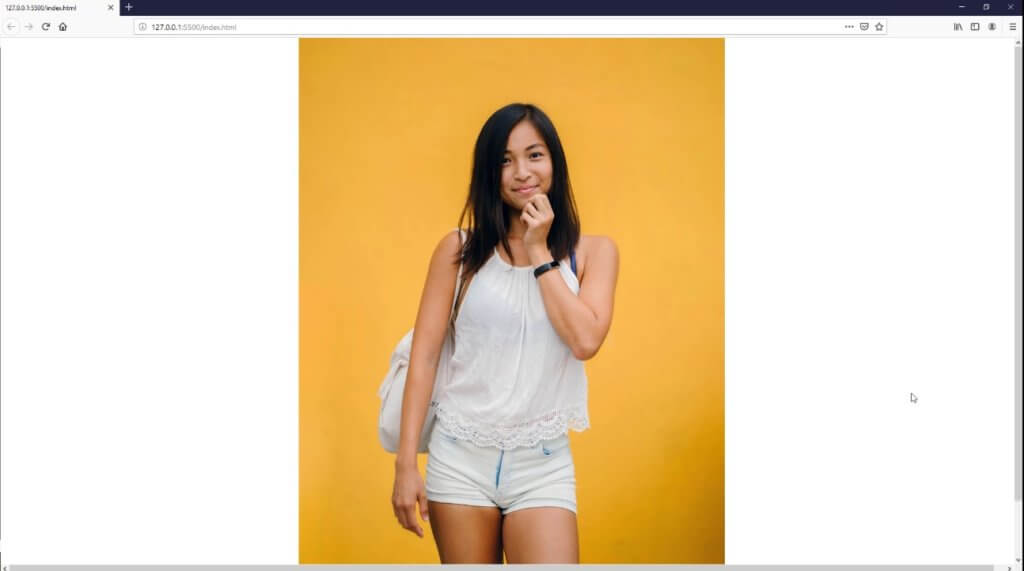```let c = document.createElement("canvas");
let img1 = new Image();

document.getElementById("image1").remove();

w = img1.width;
h = img1.height;

c.width = w;
c.height = h;
ctx = c.getContext('2d');
ctx.drawImage(img1, 0, 0);

//continue the image processing

};

img1.src = document.getElementById("image1").src;```

Next we’ll create another image object to be used as an output. We’ll try to export the current canvas image to be able to see the result. Just use toDataURL method from the canvas object and set it as output image src. Then set the size and append it to the body.

```let img2 = new Image();
img2.src = c.toDataURL("image/jpeg");
img2.width = 600;
document.body.appendChild(img2);```

Now you should see a smaller image on the page instead of the old one (as I reduced the width to 600px)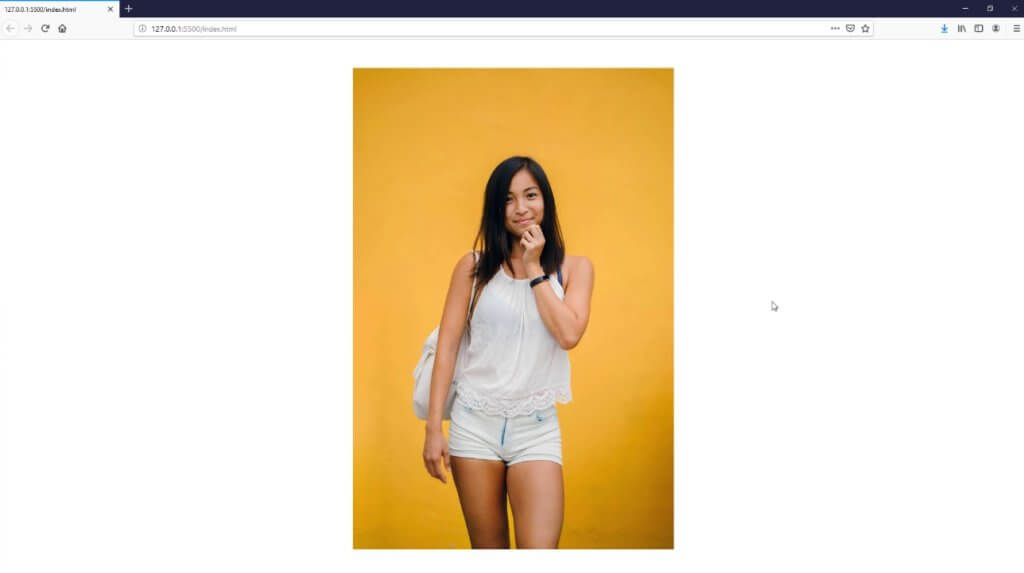Now let’s work on the pixelating process. First we’ll get the pixels array using getImageData from canvas 2D context

`let pixelArr = ctx.getImageData(0, 0, w, h).data;`

Then we’ll set the sample size to define how much pixelated effect would be. The larger sample, the larger the square size. So let’s set it to 40 pixels.

`let sample_size = 40;`

Next we’re going to loop through the pixel array. But first we need to understand the structure.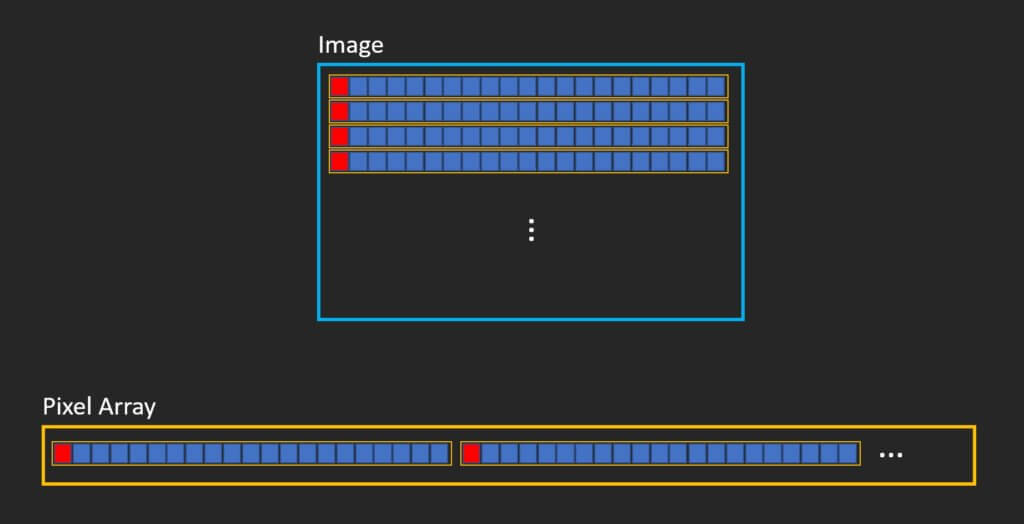The array has only single dimension so it starts with the first pixel of the first row, follow by the next pixels in the same row. then start over with the next row repeatedly until the end.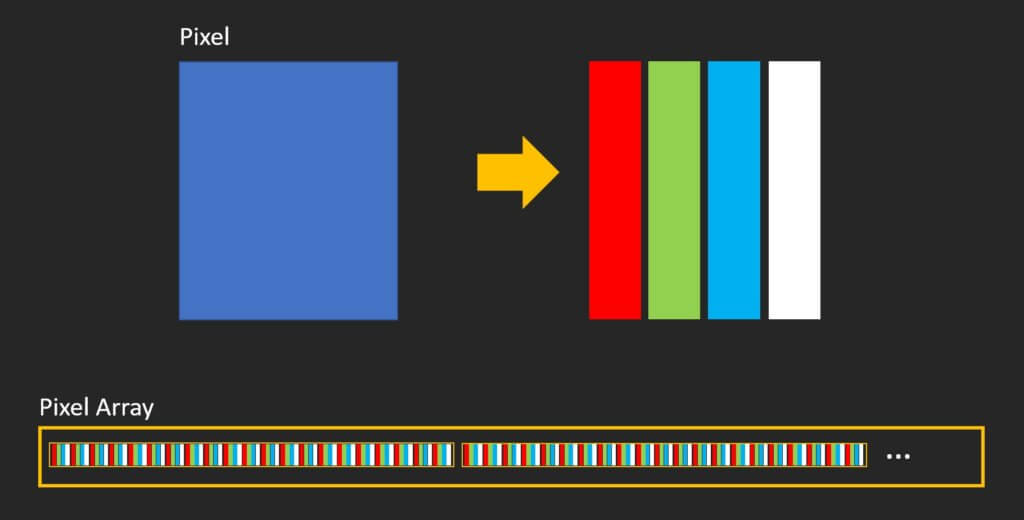Now each pixel has 4 data. The first three is RGB value and the the last one is alpha or transparency. So the array size will be 4 times of the number of pixels.

Now let’s create the loop. We’ll increase the count by the sample size. Then we’ll get the pixel index position. Don’t forget to multiply it by 4.

```for (let y = 0; y < h; y += sample_size) {
for (let x = 0; x < w; x += sample_size) {
let p = (x + (y*w)) * 4;
}
}```

Then we’ll take the RGB and alpha data from the first pixel in the sample size to represent the entire square and paint it on the canvas.

```ctx.fillStyle = "rgba(" + pixelArr[p] + "," + pixelArr[p + 1] + "," + pixelArr[p + 2] + "," + pixelArr[p + 3] + ")";
ctx.fillRect(x, y, sample_size, sample_size);```

And here is the result.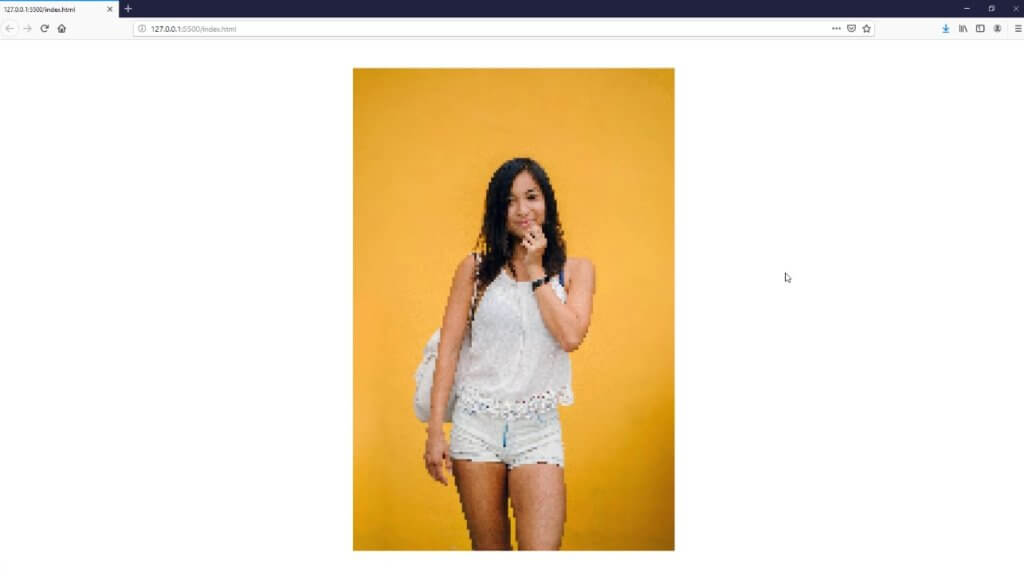You can increase the square size by adjusting the sample size.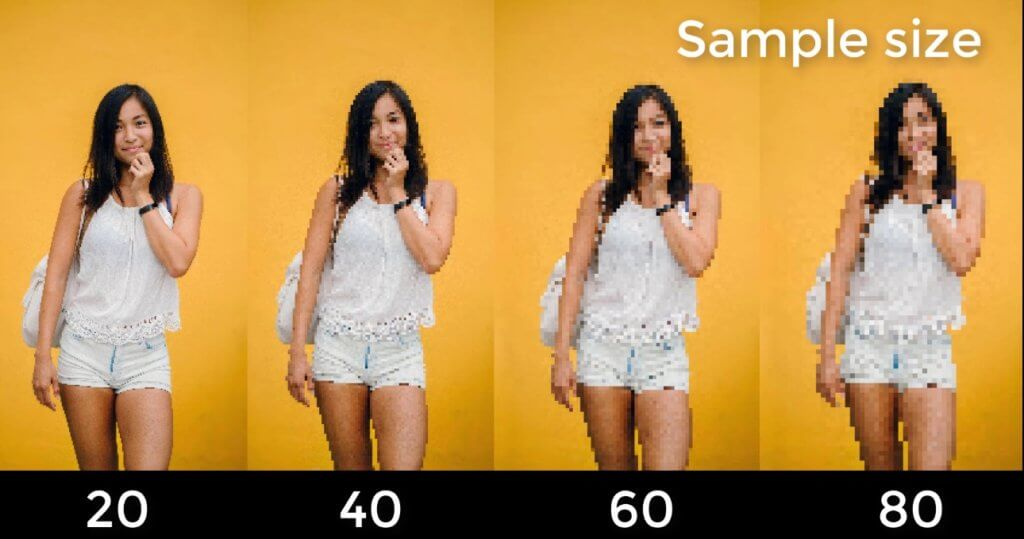And that’s all for this tutorial. If you love this post and want to see more development tips and tutorial. Don’t forget to subscribe our channel to stay tune for weekly update.

Written By

## One comment

1.Anthony E. Alvarez says:

Hello,

Great topic and video. Question: how to make a portion of the image pixelated instead of the whole image? For example: how to make only the face pixelated and not the entire of the image?

Thank you.

error: